# RSI Strategy

## Description

RSI Strategy is build to gain profit on buying / selling shares in a specific market conditions. RSI Strategy calculates two values:

• Average gain – weighted average of gains
• Average loss – weighted average of loses

This two values are used to compute a Relative Strength Index (RSI):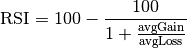Moreover, there are two RSI values given: overbought (normally around 70) and oversold (normally around 30) which are used in a process of making a decision if a strategy should sell or buy an asset. In a process of computing both averages we use some number of last price values (= Number of Periods; normally around 20). Consider a price of the last trade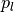and the previous one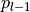. Gain has positive value when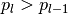, otherwise it is equal to zero. Loss can be defined in a similar way. It has positive value when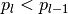, otherwise it is equal to zero.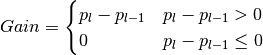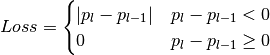RSI strategy starts decision rule, if orders should be sent after receiving Number of Periods trades.

## Parameters

PARAMETER NAME DESCRIPTION ESSENTIAL
Number of Periods Number of last ‘last trades’ events taken into account in a computation of the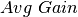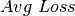Yes
Oversold Level Upper boundary of the RSI values area where market is oversold Yes
Overbought Level Lower boundary of the RSI values area where market is overbought Yes

## Conditions

### Open position

Amount One asset per order
Price Last market price
Type Price limit

If Overbought Level is crossed by the counted RSI from the top side then sell order is sent. Similarly, when the Oversold Level is crossed from the bottom side by the counted RSI the buy order is sent.

### Close position

There is no closing position condition.

### Termination

There is no strategy termination condition.

### Time frame

Strategy bases on some initial Number of periods of data collection without trading and is designed to enable adjusting both: period of data collection and its trading time span from hours to whole weeks.